# Exam-Style Questions.

## Problems adapted from questions set for previous Mathematics exams.

### 1.

GCSE Higher

$$ABC$$ is a right-angled triangle.

John has a method for finding the length of $$AC$$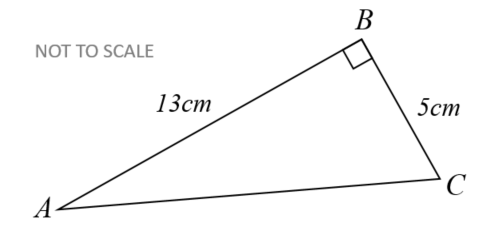$$AC^2 = AB^2 - BC^2$$ $$AC^2 = 13^2 - 5^2$$ $$AC^2 = 169 - 25$$ $$AC^2 = 144$$ $$AC = \sqrt{144}$$ $$AC = 12$$

John's answer of $$12cm$$ is not correct.

(a) What mistake has he made with his method?

(b) Show the correct method and the correct answer to 3 significant figures.

### 2.

GCSE Higher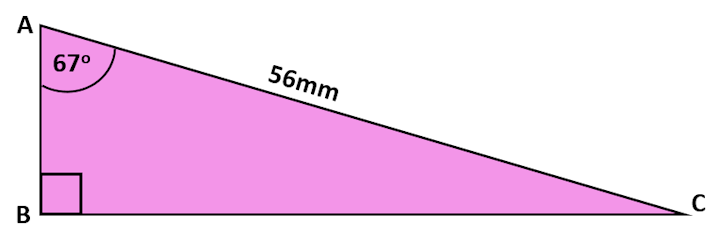The diagram (not drawn to scale) shows a right-angled triangle.

Calculate the length of side BC to three significant figures.

### 3.

GCSE Higher

An isosceles triangle shaped frame is made from four pieces of metal. The frame has a height of 8 metres and a base of length 12 metres.

The weight of the metal is 2.5kg per metre. Calculate the total weight of the metal in the frame.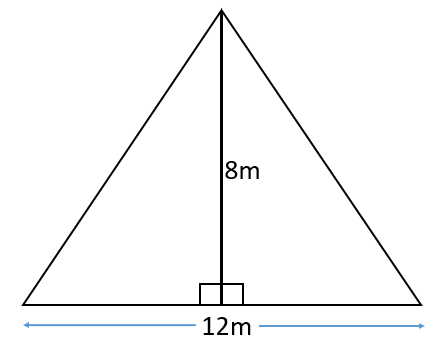### 4.

GCSE Higher

Work out the value of $$x$$, the length in centimetres of one of the sides of this right-angled triangle.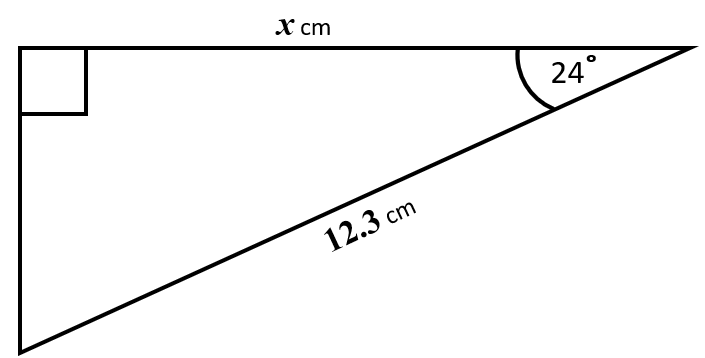### 5.

GCSE Higher

ABC is a right-angled triangle as shown in the diagram below. Calculate the length of AB giving your answer correct to three significant figures.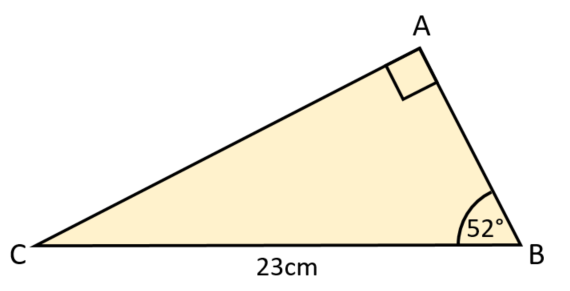### 6.

GCSE Higher

An arborist sights the top of a tree using a clinometer and reads the angle of elevation to be 29o. Her clinometer is 28 metres from the base of the tree and is on a tripod making it 1.5 metres above ground level.

This diagram is not drawn to scale.Calculate the full height of the tree.

### 7.

GCSE Higher

The diagram shows a right-angled triangle and a semicircle. The straight side of the semicircle is the same length as the longest side of the triangle.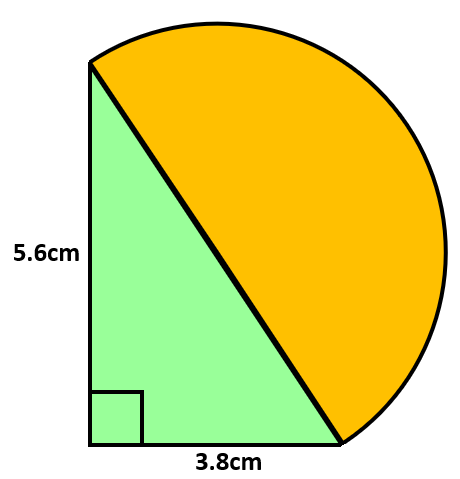Work out the area of the semicircle.

You must show all your working.

### 8.

GCSE Higher

The diagram shows a trapezium where the sides AC and BD are parallel.Calculate the length of side CD

### 9.

GCSE Higher

Four copies of a green right-angled triangle are used to enclose a yellow square.Find the area of the yellow square if the longest side of the green triangle is of length $$a$$ cm and the shortest side is $$b$$ cm.

### 10.

GCSE Higher

Taptaya Park Tower is over two hundred metres tall. There is a zip wire from the observation deck to a point on the ground 550 metres away in a northerly direction.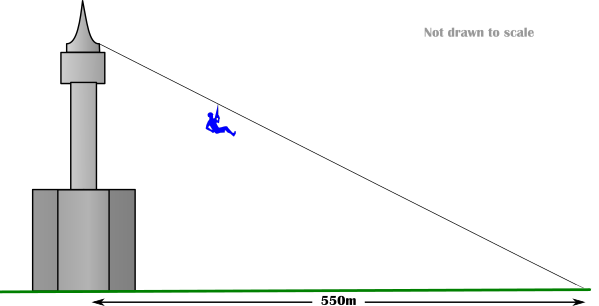(a) If the beginning of the zip wire is 210 metres above the ground find the angle of depression of the end point of the zip wire as seen from the beginning.

It is decided that the zip wire is slightly too steep so the end point is moved so that it is now further away.

(b) Find the horizontal distance if the angle of elevation of the beginning of the zip wire as seen from the end is now 18o.

### 11.

GCSE Higher

Triangle ABC has a right angle at C.

Angle BAC = 54°.

AB = 22.6 cm.

Calculate the length of BC to three significant figures.

### 12.

IB Studies

Neung is at Bangkok's Suvarnabhumi Airport watching the planes take off. He observes a plane that is at an angle of elevation of 25o from where he is standing at point N. The plane is at a height of 390 metres as can be seen in the following diagram (not to scale).(a) Calculate the horizontal distance, NH, of the plane from Neung. Give your answer to the nearest metre.

The plane took off from a point T, which is 230 metres from where Neung is standing, as shown in the following diagram (not to scale).(b) Calculate the angle ATH, the take-off angle of the plane to the nearest degree.

### 13.

GCSE Higher

A square has sides of length $$x$$ cm.

The equilateral triangle next to it has sides which are each 3cm more than the length of a side of the square.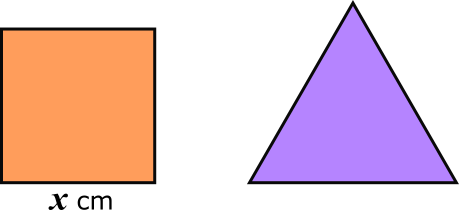(a) Find the perimeter of the square if it is equal to the perimeter of the triangle.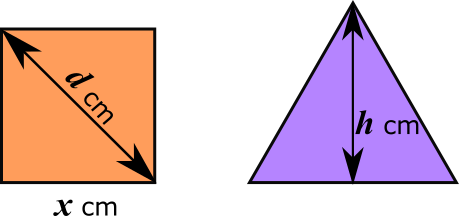The diagram above show the same square and triangle.

The length of the diagonal of the square is $$d$$ cm and the height of the triangle is $$h$$ cm.

(b) Which has the greater value, $$d$$ or $$h$$?

### 14.

GCSE Higher

(a) Write down the exact value of $$\tan 60^o$$.

(b) Find the exact area of this triangle.### 15.

GCSE Higher

Rectangle ABCD is the horizontal base of a trapezoidal prism ABCDEFGH.

EF is parallel to AB. BC = 20cm, DC = 150mm, GC = 74mm, HG = 86mm and AE = BF.(a) Find the size of angle ABF.

(b) Show that FD = 242 mm correct to 3 significant figures.

### 16.

GCSE Higher

The diagram shows a rectangular-based pyramid, TABCD (not drawn to scale).

The horizontal base ABCD has sides of lengths 11m and 15m. The centre of the base of the pyramid is M.

Angle TMC is 90° and angle TCM is 70°

The volume of a pyramid is $$\frac13$$ × area of base × perpendicular height. Calculate the volume of this pyramid.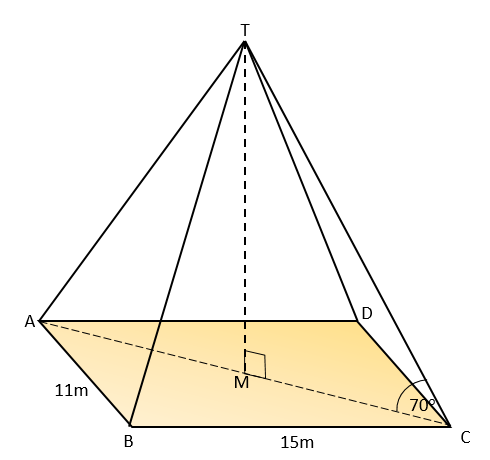### 17.

IB Studies

ABC is a triangular car park on horizontal ground. The length of AB is 90m and the length of AC is 65m. The size of angle BCA is 68o.

(a) Find the size of angle ABC.

(b) Find the area of the triangular car park.

M is the midpoint of AC.

(c) Find the length of BM.

A vertical flag pole, FB, is erected in one corner of the car park with its base at B.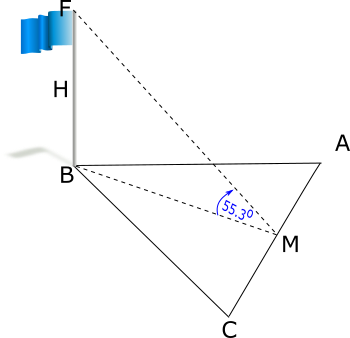The angle of elevation of F from M is 55.3o. H is the midpoint of the flag pole.

(d) Calculate the angle of elevation of H from M.

### 18.

GCSE Higher

The gradient of the line joining the origin to the point A is $$\frac12$$

The distance between A and the origin is $$\sqrt{2205}$$

What are the coordinates of A?If you would like space on the right of the question to write out the solution try this Thinning Feature. It will collapse the text into the left half of your screen but large diagrams will remain unchanged.

The exam-style questions appearing on this site are based on those set in previous examinations (or sample assessment papers for future examinations) by the major examination boards. The wording, diagrams and figures used in these questions have been changed from the originals so that students can have fresh, relevant problem solving practice even if they have previously worked through the related exam paper.

The solutions to the questions on this website are only available to those who have a Transum Subscription.

Exam-Style Questions Main Page

Search for exam-style questions containing a particular word or phrase:

To search the entire Transum website use the search box in the grey area below.